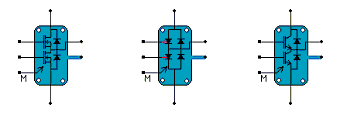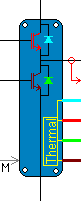• Introduction
• What is in this manual
• What is Caspoc
• User interface
• Introduction
• Starting
• Simulation
• Editing
• Viewing and printing
• Getting Started
• Basic editing
• Simulation in the time domain
• Basic User Interface Topics
• Editing
• Simulation
• Viewing
• Library
• Reports
• Project management
• Circuit and Block Diagram Components
• Introduction
• Cscript and user defined functions
• Component parameters
• Modeling Topics
• Introduction
• Power Electronics
• Semiconductors
• Electrical Machines
• Electrical drives
• Power Systems
• Mechanical Systems
• Thermal Systems
• Magnetic Circuits
• Green Energy
• Coupling to FEM
• Experimenter
• Analog hardware description language
• Embedded C code Export
• Coupling to Spice
• Small Signal Analysis
• Matlab coupling
• Tips and tricks
• Appendices

## Evaluating losses in Mosfet, IGBT, GTO.

To predict the losses in a drive system, the simulation has to run for many cycles. With the ever-increasing switching frequency, the total simulation time would be too long for a simulation employing dynamic Mosfet, IGBT or GTO models. Therefore the ideal switch model, with conduction and switching losses modeled are used to calculate the losses in the inverter.The figure above shows the modules for the fast loss prediction models. Besides the electrical nodes, each module has a thermal node that can be connected to a thermal model. In these models the turn on and turn off times can be defined from which the switching losses are calculated.

For the IGBT the IGBTMODULE4 has 4 seperate thermal nodes, one for every component.The thermal node has to be connected to a thermal model. The conduction and switching losses are predicted in this model. The conduction losses are calculated from the on-state volatge drop and the current through the device. The switching losses are predicted depending on the voltage accros and the curent through the device. The device parameters are depending on the temperature on the thermmal node. This block can also be used to model the Mosfet, wher yo usimply put the on-state voltage drop to zero.

For the IGBT, both VCEon and RCEon are temperature dependent. The switching losses are given in the manufacturer data-sheet and are specified for 25� Celsius and 125� Celsius. The junction temperature is simulated during the simulation and is used to adapt the parameters for the semiconductors.
Using the fast loss prediction model enables the prediction of the system behavior and prediction of the losses of the component. In an IGBT, the temperature dependent VCEon and RCEon model the conduction losses. The switching losses are calculated from the data-sheet parameters Eon and Eoff. The temperature on the heat sink is dependent on the losses. The losses are temperature dependent because VCEon and RCeon are temperature dependent.

Losses and Thermal simulation
The IGBTMODULE4 model has four thermal connections that have to be connected to a heat sink model. The temperature rise due to the conduction, switching and reverse recovery losses is modeled on this connection. Note that colors indicate which thermal node belongs to which component.
A heat sink is build from the components found in components/library/Heatsink The parameter Rth and Cth model the thermal model from junction to case. The initial temperature of the junction is modeled by the parameter Tth0. If a more detailed thermal model for the junction to case thermal path has to be build, Rth and Cth simply model the first chip-layer and the following layers are modeled by subsequent thermal models.

Overview of the parameters
The parameters for the IGBTMODULE4 are summarized in the following table.

Nominal Parameters
 Parameter Default Function Vnom 600 Nominal voltage for which the Eon, Eoff and Err values are given Inom 100 Nominal current for which the Eon, Eoff and Err values are given

IGBT Parameters
 Parameter Default Function Eoff25 50mJ Turn off energy loss at 25 degrees Celcius Eoff125 50mJ Turn off energy loss at 125 degrees Celcius Eon25 50mJ Turn on energy loss at 25 degrees Celcius Eon125 50mJ Turn on energy loss at 125 degrees Celcius Vce25 1 On-state voltage drop at 25 degrees Celcius Vce125 1 On-state voltage drop at 125 degrees Celcius Rce25 25m On-state resistance at 25 degrees Celcius Rce125 25m On-state resistance at 125 degrees Celcius

Diode Parameters
 Parameter Default Function Err25 50mJ Reverse recovery energy loss at 25 degrees Celcius Err125 50mJ Reverse recovery energy loss at 125 degrees Celcius Vd25 0.5 On-state voltage drop at 25 degrees Celcius Vd125 0.5 On-state voltage drop at 25 degrees Celcius Rd25 10m On-state resistance at 25 degrees Celcius Rd125 10m On-state resistance at 125 degrees Celcius

IGBTModule4x
The IGBTModule4x can be used if detailed information is available regarding the dependency of the losses on load current and gate resistance.
The are two files required, with the following format:

E_ic.dat:
 ic1 EonT1 EonT2 EoffT1 EoffT2 ErecT1 ErecT2 ic2 EonT1 EonT2 EoffT1 EoffT2 ErecT1 ErecT2 . . icn EonT1 EonT2 EoffT1 EoffT2 ErecT1 ErecT2 
E_Rg.dat:
 Rg1 Eon Eoff Erec Rg2 Eon Eoff Erec . . Rgn Eon Eoff Erec